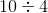# Computer Science : Int

## Example Questions

### Example Question #1 : Primitive Data Types

int x = 10;

int y = 4;



int z = x / y;

What is the value of z?Explanation:

There are two reasons why this will not produce the expected output, 2.5:

1. When dividing by two integer operands, the result will be returned as an int. Solve this by casting either of the operands (or the entire expression) to the float or double type.
2. When storing a floating-point value in an integer variable, the decimal is truncated (discarded, cut off, not considered). Even ifwas configured as suggested in step 1, and returned 2.5, the value 2 would be the value stored in z. Solve this value by declaring z as a float.

### Example Question #1 : Primitive Data Types

How do we set a method to return an Int in Swift(iOS)?

method->Int {}

func method() -> Int {}

func method->Int{}

func() -> Int{}

func method() -> Int {}

Explanation:

In Swift, all methods must first say what they are. They are functions, so they are prefixed with func. Next, methods must have a name. In this case, we named it method. All methods need to specify parameters, even if there are no parameters. So, method() i.e. no parameters. Finally, we wanted to return an Int. So we set the return type using -> Int.

### Example Question #1 : Primitive Data Types

How do we set a method to return an Int in Swift(iOS)?

method->Int {}

func method() -> Int {}

func method->Int{}

func() -> Int{}